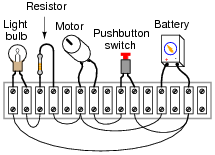# Simple Circuits

## Basic Electricity

• #### Question 1

Shown here is the schematic symbol for a resistor: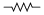What is the purpose of a resistor? What function does it perform? Also, draw an illustration of what a real resistor looks like.

• #### Question 2

Resistors are sometimes represented in electrical and electronic schematic diagrams by a symbol other than this:Draw this other symbol next to the one shown above.

• #### Question 3

What will this voltmeter register when connected to a battery as shown (assume a battery voltage of 6 volts)? Explain your answer.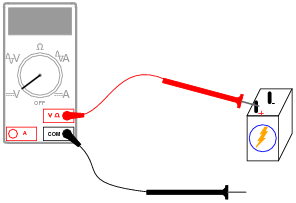• #### Question 4

What will this voltmeter register when connected to a battery as shown (assume a battery voltage of 6 volts)? Explain your answer.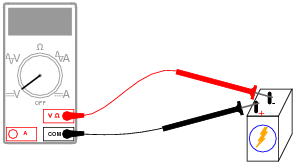• #### Question 5

Determine what these four voltmeters (A, B, C, D) will register when connected to this circuit in the following positions (assume a battery voltage of 6 volts):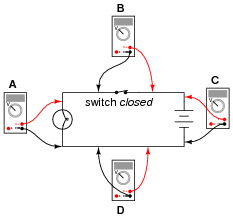• Voltmeter A =
• Voltmeter B =
• Voltmeter C =
• Voltmeter D =
• #### Question 6

Determine what these four voltmeters (A, B, C, D) will register when connected to this circuit in the following positions (assume a battery voltage of 6 volts):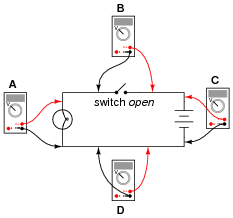• Voltmeter A =
• Voltmeter B =
• Voltmeter C =
• Voltmeter D =
• #### Question 7

Shown here is a circuit constructed on a PCB (a “Printed Circuit Board”), with copper “traces” serving as wires to connect the components together: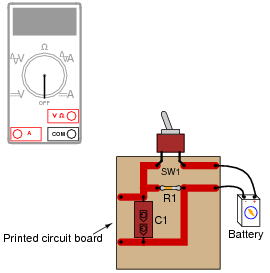How would the multimeter be used to measure the voltage across the component labeled “R1” when energized? Include these important points in your answer:

• The configuration of the multimeter (selector switch position, test lead jacks)
• The connections of the meter test leads to the circuit
• The state of the switch on the PCB (open or closed)
• #### Question 8

What difference will it make if the switch is located in either of these two alternate locations in the circuit?• #### Question 9

Identify the following types of switches, according to the number of “poles” and “throws” each switch has:• #### Question 10

Identify the following types of switches, according to their style of actuation (how each switch is physically operated):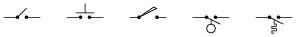• #### Question 11

What type of switch is represented by this schematic symbol?• #### Question 12

What positions do the switches have to be in for the light bulb to receive power?• #### Question 13

Electric motors of the permanent magnet design are very simple to reverse: just switch the polarity of the DC power to the motor, and it will spin the other direction:Complete this schematic diagram, showing how a DPDT switch may be placed in this circuit to reverse the motor’s direction of rotation without the need to disconnect and re-connect wires:• #### Question 14

What will the light bulb do when the switch is open, and when the switch is closed?• #### Question 15

Examine this schematic diagram:Now, without moving the following components, show how they may be connected together with wires to form the same circuit depicted in the schematic diagram above: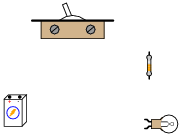• #### Question 16

In this circuit, where would you expect to measure full battery voltage (between what pairs of test points)?• #### Question 17

In this circuit, where would you not expect to measure significant voltage (between what pairs of test points)?• #### Question 18

Suppose this battery and light bulb circuit failed to work. Using nothing but a voltmeter, how would you check the circuit to determine where the problem is located? Note: the letters indicate “test points” along the wiring where you may probe with the circuit with your voltmeter.• #### Question 19

Suppose this battery and light bulb circuit failed to work:Using a voltmeter, a technician measures full battery voltage between the points C and H. What does this single measurement indicate about the condition of the circuit? Be as specific as you can.

• #### Question 20

Suppose this battery and light bulb circuit failed to work:Using a voltmeter, a technician measures full battery voltage between the points C and H. The result of this single measurement indicates which half of the circuit there is a definite problem in. What would you recommend as the next voltmeter measurement to take in troubleshooting the circuit, following the same “divide in half” strategy?

• #### Question 21

Suppose this battery and light bulb circuit failed to work:Using a voltmeter, a technician measures 0 volts between the points C and H. What does this single measurement indicate about the condition of the circuit? Be as specific as you can.

• #### Question 22

Suppose this battery and light bulb circuit failed to work:Using a voltmeter, a technician measures 0 volts between the points C and H. The result of this single measurement indicates which half of the circuit there is a definite problem in. What would you recommend as the next voltmeter measurement to take in troubleshooting the circuit, following the same “divide in half” strategy?

• #### Question 23

Suppose this battery and light bulb circuit failed to work:Using nothing but a voltmeter, a technician measures voltage between the following sets of points:

• Between A and C: 0 volts
• Between D and G: 12 volts
• Between E and J: 0 volts
• Between B and E: 12 volts

From these voltage measurements, what can you tell about the condition of the battery, wiring, and light bulb? Be as specific as you can.

Challenge question: identify which of the four measurement are unnecessary in determining the precise location of the fault in this circuit.

• #### Question 24

The circuit shown here is called a “bridge rectifier,” and its purpose is to convert alternating current (from the “power-supply” unit) into direct current. Suppose you were instructed to check the continuity of the switch (SW1) mounted on the printed circuit board. What would be a fast and effective way of testing this switch’s continuity (ideally, without removing the switch from the circuit board)?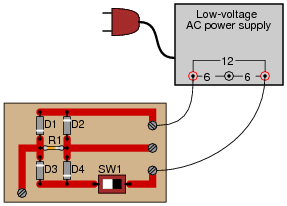• #### Question 25

In the simplest terms you can think of, define what an electrical circuit is.

• #### Question 26

Given a battery and a light bulb, show how you would connect these two devices together with wire so as to energize the light bulb: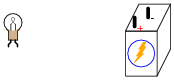• #### Question 27

Build a simple electric circuit using a battery as the electrical energy source, and a small light bulb as the electrical load (I suggest using a 6-volt “lantern” battery and a miniature incandescent light bulb rated for either 6 or 12 volts). Use “jumper” wires with metal clips at the ends to join these two electrical devices together: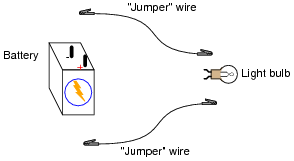After connecting the components together properly so the light bulb lights up, answer the following questions:

• What conditions must be met for the light bulb to light up?
• What happens if the circuit is “broken”?
• Does it matter where the circuit is “broken”?

Then, add a third jumper wire to the circuit so you have a ready “break” to experiment with: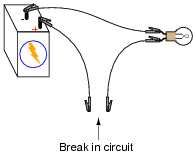Try bridging this “break” with various materials, and note whether or not the light bulb lights up:

Paper
Steel paper clip
Gold ring
Rubber eraser

Also, try touching the jumper wire ends together along their plastic exteriors, rather than at the metal “clip” ends. Does the light bulb light up when you do this?

Explain what this experiment demonstrates about the electrical conductivity of the various substances listed as well as the plastic coating of the jumper wires. Also explain why electrical wires are provided with that plastic coating, instead of being bare metal. Finally, explain what this experiment has taught you about electric circuits in general.

• #### Question 28

Examine this schematic diagram:Now, without moving the following components, show how they may be connected together with wires to form the same circuit depicted in the schematic diagram above: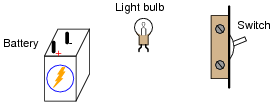• #### Question 29

Shown here is a circuit constructed on a PCB (a “Printed Circuit Board”), with copper “traces” serving as wires to connect the components together:How would the multimeter be used to measure the current through the component labeled “R1” when energized? Include these important points in your answer:

• The configuration of the multimeter (selector switch position, test lead jacks)
• The connections of the meter test leads to the circuit
• The state of the switch on the PCB (open or closed)
• #### Question 30

Why is it a very bad idea to connect an ammeter directly across a voltage source, like this?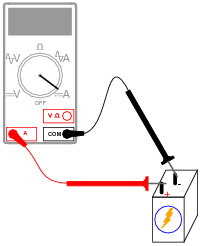• #### Question 31

What would happen if a multimeter were connected across the component labeled “C1” on this printed circuit board, as shown?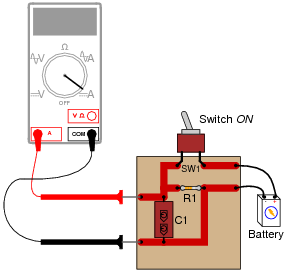• #### Question 32

Explain how an ohmmeter is able to measure the resistance of a component (in this case, a light bulb) when there is no battery or other source of power connected to it: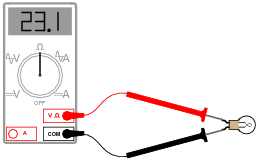Also, identify the reading you would expect the ohmmeter to indicate if the light bulb were burnt out (failed “open”).

• #### Question 33

Shown here is a circuit constructed on a PCB (a “Printed Circuit Board”), with copper “traces” serving as wires to connect the components together: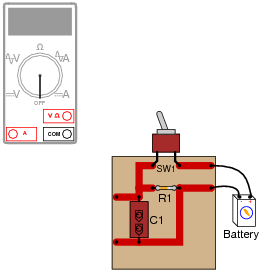How would the multimeter be used to measure the resistance of the component labeled “R1”? Include these important points in your answer:

• The configuration of the multimeter (selector switch position, test lead jacks)
• The connections of the meter test leads to the circuit
• The state of the switch on the PCB (open or closed)
• #### Question 34

In this circuit, is the light bulb lit? Why or why not?

Also, compare the relative indications of the two ammeters (which ammeter registers the greatest amount of current, and which ammeter registers the least amount of current, or do they both register the same amount of current?).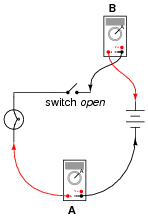• #### Question 35

In this circuit, is the light bulb lit? Why or why not?

Also, compare the relative indications of the two ammeters (which ammeter registers the greatest amount of current, and which ammeter registers the least amount of current, or do they both register the same amount of current?).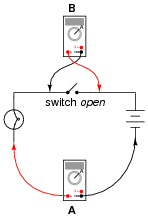• #### Question 36

In this circuit, is the light bulb lit? Why or why not?

Also, compare the relative indications of the two ammeters (which ammeter registers the greatest amount of current, and which ammeter registers the least amount of current, or do they both register the same amount of current?).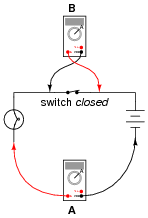• #### Question 37

Re-draw this circuit in the form of a schematic diagram: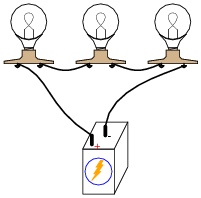• #### Question 38

What would happen if three 6-volt light bulbs were connected as shown to a 6-volt battery? How would their brightnesses compare to just having a single 6-volt light bulb connected to a 6-volt battery?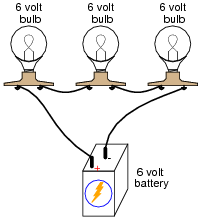• #### Question 39

Qualitatively compare the voltage and current for each of the three light bulbs in this circuit (assume the three light bulbs are absolutely identical):• #### Question 40

What difference will it make if the battery in this circuit is reversed in direction?• #### Question 41

From observation of this circuit (with components attached to a “terminal strip”), draw an appropriate schematic diagram: